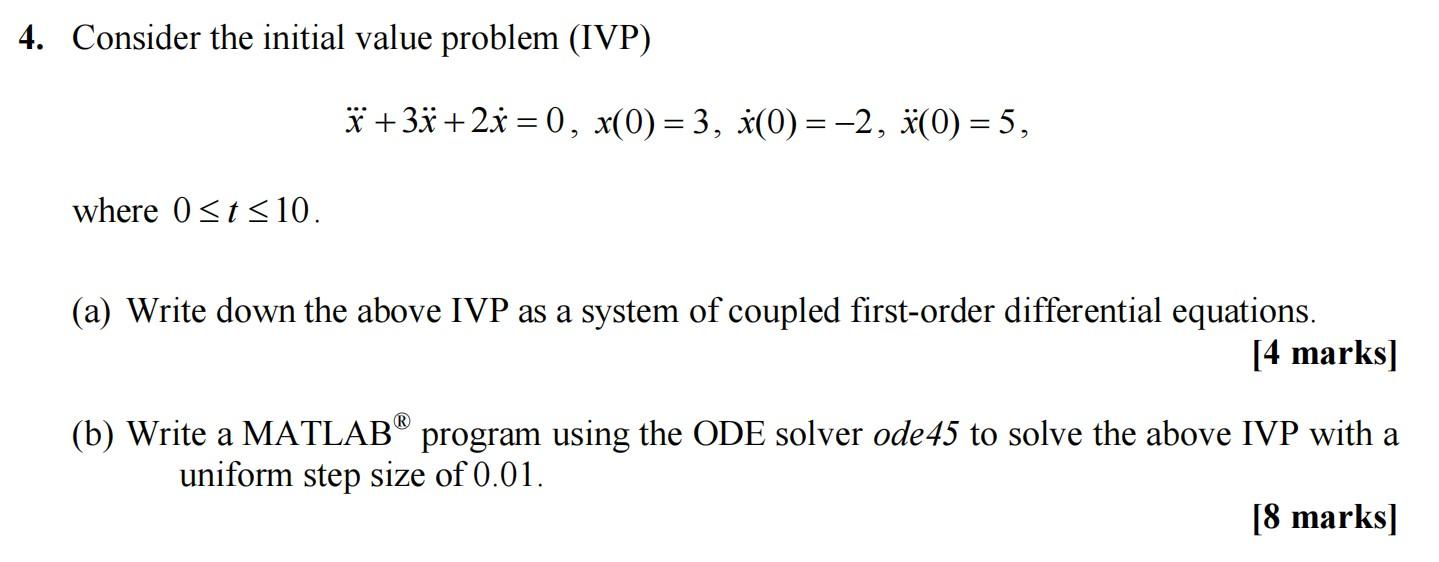# Question   Dear expert ,Please answer this Numerical methods for physicists question completely .Please explain the problem stepwise and  give explanations so that we can understand .Thank you we value your time and efforts.​​​​​​​ 4. Consider the initial value problem (IVP) $\dddot{x}+3 \ddot{x}+2 \dot{x}=0, x(0)=3, \dot{x}(0)=-2, \ddot{x}(0)=5,$ where $$0 \leq t \leq 10$$. (a) Write down the above IVP as a system of coupled first-order differential equations. [4 marks] (b) Write a MATLAB $${ }^{\circledR}$$ program using the ODE solver ode45 to solve the above IVP with a uniform step size of $$0.01$$. [8 marks]Dear expert ,Please answer this Numerical methods for physicists question completely .Please explain the problem stepwise and  give explanations so that we can understand .Thank you we value your time and efforts.​​​​​​​Transcribed Image Text: 4. Consider the initial value problem (IVP) $\dddot{x}+3 \ddot{x}+2 \dot{x}=0, x(0)=3, \dot{x}(0)=-2, \ddot{x}(0)=5,$ where $$0 \leq t \leq 10$$. (a) Write down the above IVP as a system of coupled first-order differential equations. [4 marks] (b) Write a MATLAB $${ }^{\circledR}$$ program using the ODE solver ode45 to solve the above IVP with a uniform step size of $$0.01$$. [8 marks]
Transcribed Image Text: 4. Consider the initial value problem (IVP) $\dddot{x}+3 \ddot{x}+2 \dot{x}=0, x(0)=3, \dot{x}(0)=-2, \ddot{x}(0)=5,$ where $$0 \leq t \leq 10$$. (a) Write down the above IVP as a system of coupled first-order differential equations. [4 marks] (b) Write a MATLAB $${ }^{\circledR}$$ program using the ODE solver ode45 to solve the above IVP with a uniform step size of $$0.01$$. [8 marks]